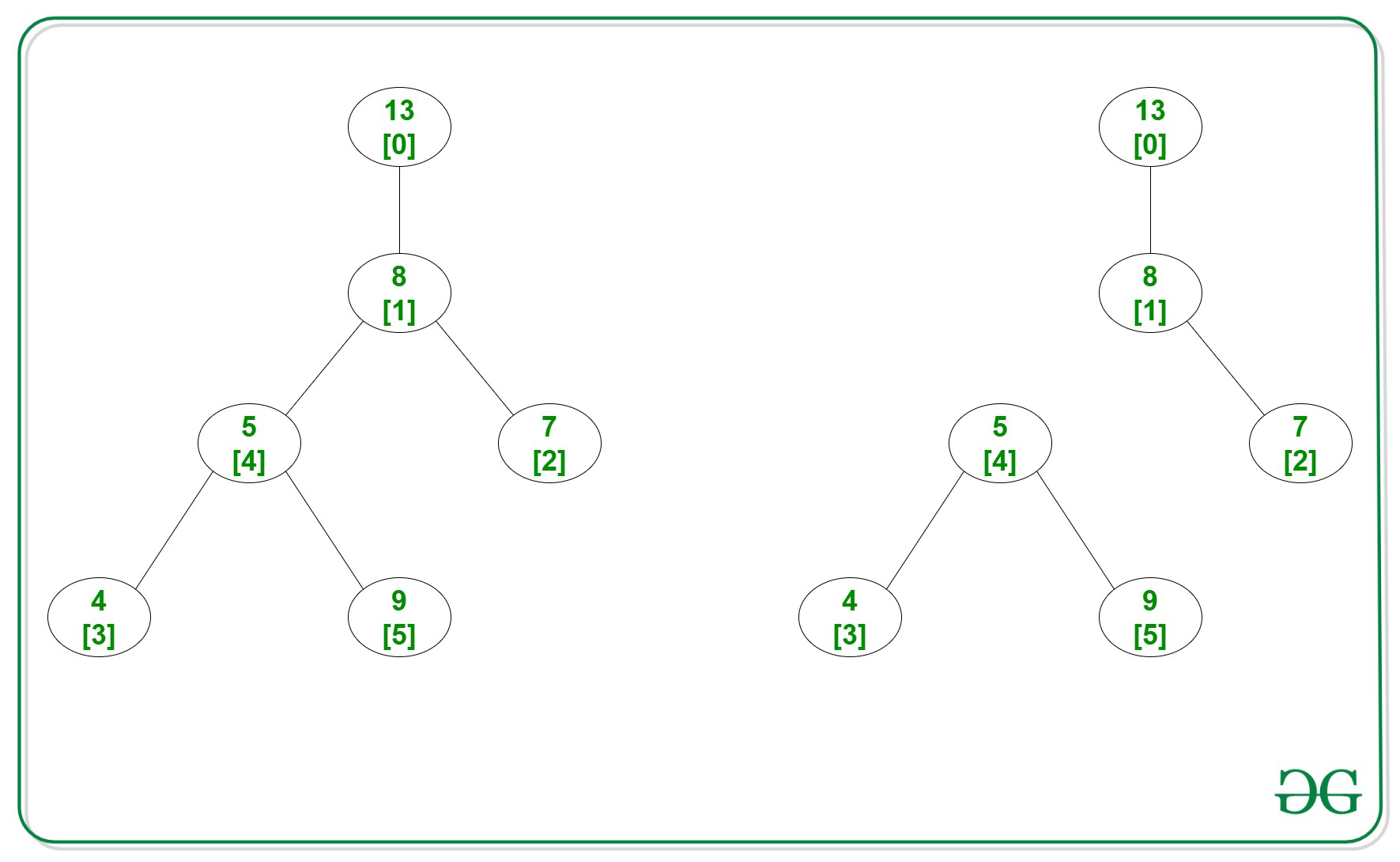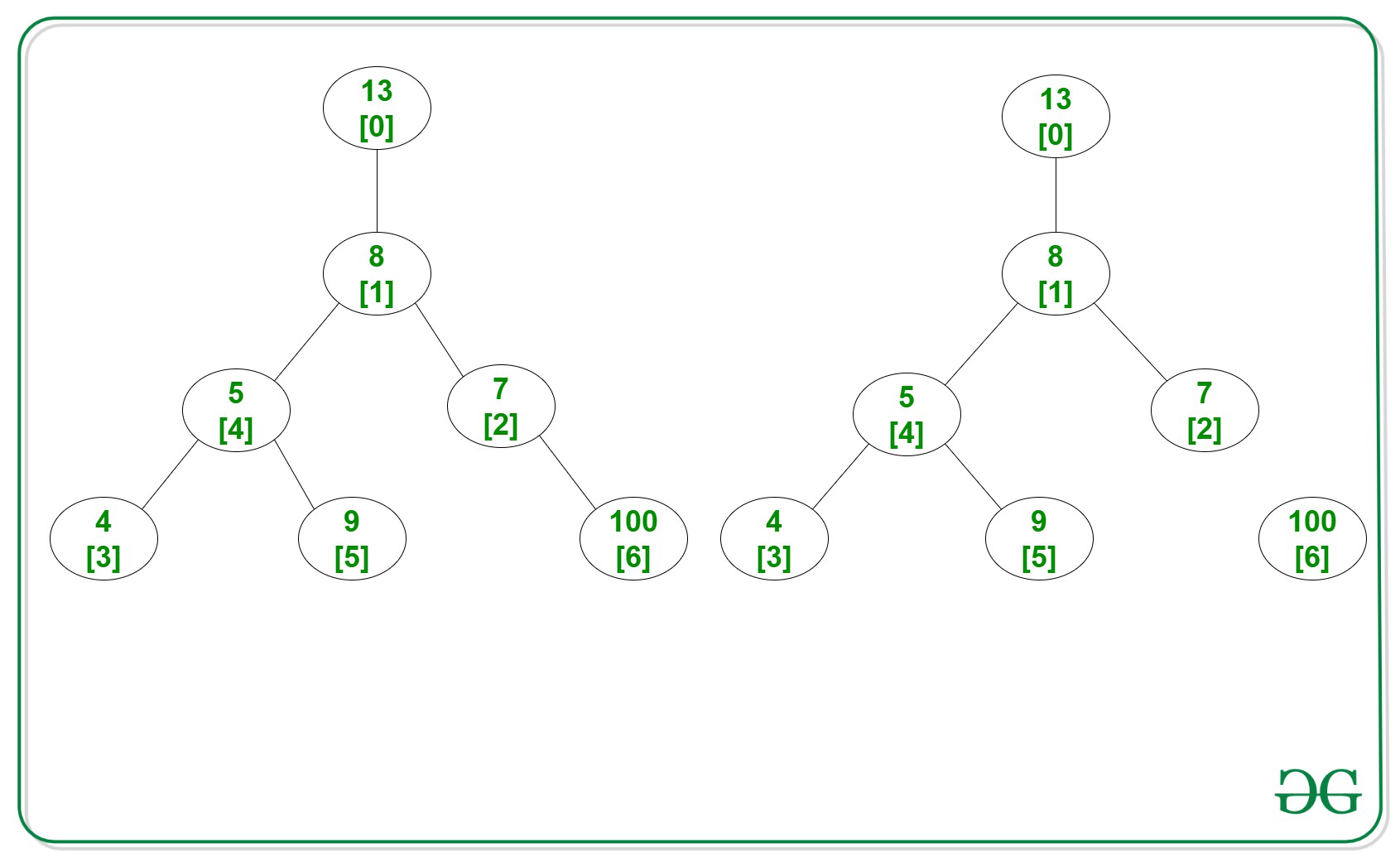Related Articles

# Maximum cost of splitting given Binary Tree into two halves

• Difficulty Level : Medium
• Last Updated : 21 Jun, 2021

Given a Binary Tree with N nodes valued 0 to N – 1 and N-1 edges and an array arr[] consisting of values of edges, the task is to find the maximum cost of splitting the tree into two halves.

The cost of splitting a tree is equal to the product of sum of node values of the splitted subtrees.

Examples:

Input: N = 6, arr[] = {13, 8, 7, 4, 5, 9}, Edges[][] = {{0, 1}, {1, 2}, {1, 4}, {3, 4}, {4, 5}}
Output: 504
Explanation:
Below is the given tree and resultant tree after removing the edge:Remove the edge between 1st and 4th, then
t1 = valueat + valueat + valueat = 13 + 8 + 7
t1 = valueat + valueat + valueat = 4 + 5 + 9
t1*t2 = (13 + 8 + 7) * (4 + 5 + 9) = 504

Input: N = 7, arr[]= {13, 8, 7, 4, 5, 9, 100}, Edges[][] = { {0, 1}, {1, 2}, {1, 4}, {3, 4}, {4, 5}, {2, 6}}
Output: 4600
Explanation:
Below is the given tree and resultant tree after removing the edge:Remove the edge between 2nd and 6th, then
t1 = valueat + valueat + valueat + valueat + valueat + valueat= 13 + 8 + 7 + 4 + 5 + 9
t2 = valueat = 100
t1*t2 = (13 + 8 + 7 + 5 + 4 + 9) * (100) = 4600

Approach: The idea is to traverse the given tree and try to break the tree at every possible edge and then find the maximum cost of splitting at that edges. After all the above steps print the maximum cost among all the splitting. Below are the steps:

1. All the edges are stored using the adjacency list edges and values at each node is stored in the given array arr[].
2. For the current-node, find the sum of values in its descendants including itself.
3. Suppose if the edge between the current node and its parent is removed then two trees can be formed.
4. Now, calculate values of t1, t2, and check the product of t1 and t2 is maximum or not.
5. Repeat this process recursively to all the child-nodes of current-node.

Below is the implementation of the above approach:

## C++

 `// C++ program for the above approach``#include ``using` `namespace` `std;` `// To store the results and sum of``// all nodes in the array``int` `ans = 0, allsum = 0;` `// To create adjacency list``vector<``int``> edges;` `// Function to add edges into the``// adjacency list``void` `addedge(``int` `a, ``int` `b)``{``    ``edges[a].push_back(b);``    ``edges[b].push_back(a);``}` `// Recursive function that calculate``// the value of the cost of splitting``// the tree recursively``void` `findCost(``int` `r, ``int` `p, ``int` `arr[])``{``    ``int` `i, cur;` `    ``for` `(i = 0; i < edges[r].size();``         ``i++) {` `        ``// Fetch the child of node-r``        ``cur = edges[r].at(i);` `        ``// Neglect if cur node is parent``        ``if` `(cur == p)``            ``continue``;` `        ``findCost(cur, r, arr);` `        ``// Add all values of nodes``        ``// which are decendents of r``        ``arr[r] += arr[cur];``    ``}` `    ``// The two trees formed are rooted``    ``// at 'r' with its decendents``    ``int` `t1 = arr[r];``    ``int` `t2 = allsum - t1;` `    ``// Check and replace if current``    ``// product t1*t2 is large``    ``if` `(t1 * t2 > ans) {``        ``ans = t1 * t2;``    ``}``}` `// Function to find the maximum cost``// after splitting the tree in 2 halves``void` `maximumCost(``int` `r, ``int` `p,``                 ``int` `N, ``int` `M,``                 ``int` `arr[],``                 ``int` `Edges[])``{``    ``// Find sum of values in all nodes``    ``for` `(``int` `i = 0; i < N; i++) {``        ``allsum += arr[i];``    ``}` `    ``// Traverse edges to create``    ``// adjacency list``    ``for` `(``int` `i = 0; i < M; i++) {``        ``addedge(Edges[i],``                ``Edges[i]);``    ``}` `    ``// Function Call``    ``findCost(r, p, arr);``}` `// Driver Code``int` `main()``{``    ``int` `a, b, N = 6;` `    ``// Values in each node``    ``int` `arr[] = { 13, 8, 7, 4, 5, 9 };` `    ``int` `M = 5;` `    ``// Given Edges``    ``int` `Edges[] = { { 0, 1 }, { 1, 2 },``                       ``{ 1, 4 }, { 3, 4 },``                       ``{ 4, 5 } };` `    ``maximumCost(1, -1, N, M, arr, Edges);` `    ``cout << ans;``    ``return` `0;``}`

## Java

 `// Java program for the above approach``import` `java.util.*;``class` `GFG{`` ` `// To store the results and sum of``// all nodes in the array``static` `int` `ans = ``0``, allsum = ``0``;`` ` `// To create adjacency list``static` `Vector []edges = ``new` `Vector[``100001``];`` ` `// Function to add edges into the``// adjacency list``static` `void` `addedge(``int` `a, ``int` `b)``{``    ``edges[a].add(b);``    ``edges[b].add(a);``}`` ` `// Recursive function that calculate``// the value of the cost of splitting``// the tree recursively``static` `void` `findCost(``int` `r, ``int` `p, ``int` `arr[])``{``    ``int` `i, cur;``    ``for` `(i = ``0``; i < edges[r].size(); i++)``    ``{``        ``// Fetch the child of node-r``        ``cur = edges[r].get(i);`` ` `        ``// Neglect if cur node is parent``        ``if` `(cur == p)``            ``continue``;`` ` `        ``findCost(cur, r, arr);`` ` `        ``// Add all values of nodes``        ``// which are decendents of r``        ``arr[r] += arr[cur];``    ``}`` ` `    ``// The two trees formed are rooted``    ``// at 'r' with its decendents``    ``int` `t1 = arr[r];``    ``int` `t2 = allsum - t1;`` ` `    ``// Check and replace if current``    ``// product t1*t2 is large``    ``if` `(t1 * t2 > ans)``    ``{``        ``ans = t1 * t2;``    ``}``}`` ` `// Function to find the maximum cost``// after splitting the tree in 2 halves``static` `void` `maximumCost(``int` `r, ``int` `p,``                        ``int` `N, ``int` `M,``                        ``int` `arr[],``                        ``int` `Edges[][])``{``    ``// Find sum of values in all nodes``    ``for` `(``int` `i = ``0``; i < N; i++)``    ``{``        ``allsum += arr[i];``    ``}`` ` `    ``// Traverse edges to create``    ``// adjacency list``    ``for` `(``int` `i = ``0``; i < M; i++)``    ``{``        ``addedge(Edges[i][``0``],``                ``Edges[i][``1``]);``    ``}`` ` `    ``// Function Call``    ``findCost(r, p, arr);``}`` ` `// Driver Code``public` `static` `void` `main(String[] args)``{``    ``int` `a, b, N = ``6``;`` ` `    ``// Values in each node``    ``int` `arr[] = {``13``, ``8``, ``7``, ``4``, ``5``, ``9``};`` ` `    ``int` `M = ``5``;`` ` `    ``// Given Edges``    ``int` `Edges[][] = {{``0``, ``1``}, {``1``, ``2``},``                     ``{``1``, ``4``}, {``3``, ``4``},``                     ``{``4``, ``5``}};``    ``for` `(``int` `i = ``0``; i < edges.length; i++)``        ``edges[i] = ``new` `Vector();``    ``maximumCost(``1``, -``1``, N, M, arr, Edges);``    ``System.out.print(ans);``}``}`` ` `// This code is contributed by Amit Katiyar`

## Python3

 `# Python3 program for the above approach` `# To store the results and sum of``# all nodes in the array``ans ``=` `0``allsum ``=` `0` `# To create adjacency list``edges ``=` `[[] ``for` `i ``in` `range``(``100001``)]` `# Function to add edges into the``# adjacency list``def` `addedge(a, b):``    ` `    ``global` `edges``    ``edges[a].append(b)``    ``edges[b].append(a)` `# Recursive function that calculate``# the value of the cost of splitting``# the tree recursively``def` `findCost(r, p, arr):``    ` `    ``global` `edges``    ``global` `ans``    ``global` `allsum``    ``i ``=` `0``    ` `    ``for` `i ``in` `range``(``len``(edges[r])):``        ` `        ``# Fetch the child of node-r``        ``cur ``=` `edges[r][i]` `        ``# Neglect if cur node is parent``        ``if` `(cur ``=``=` `p):``            ``continue` `        ``findCost(cur, r, arr)` `        ``# Add all values of nodes``        ``# which are decendents of r``        ``arr[r] ``+``=` `arr[cur]` `    ``# The two trees formed are rooted``    ``# at 'r' with its decendents``    ``t1 ``=` `arr[r]``    ``t2 ``=` `allsum ``-` `t1` `    ``# Check and replace if current``    ``# product t1*t2 is large``    ``if` `(t1 ``*` `t2 > ans):``        ``ans ``=` `t1 ``*` `t2` `# Function to find the maximum cost``# after splitting the tree in 2 halves``def` `maximumCost(r, p, N, M, arr, Edges):``    ` `    ``global` `allsum``    ` `    ``# Find sum of values in all nodes``    ``for` `i ``in` `range``(N):``        ``allsum ``+``=` `arr[i]` `    ``# Traverse edges to create``    ``# adjacency list``    ``for` `i ``in` `range``(M):``        ``addedge(Edges[i][``0``], Edges[i][``1``])` `    ``# Function Call``    ``findCost(r, p, arr)` `# Driver Code``if` `__name__ ``=``=` `'__main__'``:``    ` `    ``N ``=` `6` `    ``# Values in each node``    ``arr ``=` `[ ``13``, ``8``, ``7``, ``4``, ``5``, ``9` `]` `    ``M ``=` `5` `    ``# Given Edges``    ``Edges ``=` `[ [ ``0``, ``1` `], [ ``1``, ``2` `],``              ``[ ``1``, ``4` `], [ ``3``, ``4` `],``              ``[ ``4``, ``5` `] ]` `    ``maximumCost(``1``, ``-``1``, N, M, arr, Edges)` `    ``print``(ans)` `# This code is contributed by ipg2016107`

## C#

 `// C# program for the above approach``using` `System;``using` `System.Collections.Generic;``class` `GFG{`` ` `// To store the results and sum of``// all nodes in the array``static` `int` `ans = 0, allsum = 0;`` ` `// To create adjacency list``static` `List<``int``> []edges = ``new` `List<``int``>;`` ` `// Function to add edges into the``// adjacency list``static` `void` `addedge(``int` `a, ``int` `b)``{``    ``edges[a].Add(b);``    ``edges[b].Add(a);``}`` ` `// Recursive function that calculate``// the value of the cost of splitting``// the tree recursively``static` `void` `findCost(``int` `r, ``int` `p, ``int` `[]arr)``{``    ``int` `i, cur;``    ``for` `(i = 0; i < edges[r].Count; i++)``    ``{``        ``// Fetch the child of node-r``        ``cur = edges[r][i];`` ` `        ``// Neglect if cur node is parent``        ``if` `(cur == p)``            ``continue``;`` ` `        ``findCost(cur, r, arr);`` ` `        ``// Add all values of nodes``        ``// which are decendents of r``        ``arr[r] += arr[cur];``    ``}`` ` `    ``// The two trees formed are rooted``    ``// at 'r' with its decendents``    ``int` `t1 = arr[r];``    ``int` `t2 = allsum - t1;`` ` `    ``// Check and replace if current``    ``// product t1*t2 is large``    ``if` `(t1 * t2 > ans)``    ``{``        ``ans = t1 * t2;``    ``}``}`` ` `// Function to find the maximum cost``// after splitting the tree in 2 halves``static` `void` `maximumCost(``int` `r, ``int` `p,``                        ``int` `N, ``int` `M,``                        ``int` `[]arr, ``int` `[, ]Edges)``{``    ``// Find sum of values in all nodes``    ``for` `(``int` `i = 0; i < N; i++)``    ``{``        ``allsum += arr[i];``    ``}`` ` `    ``// Traverse edges to create``    ``// adjacency list``    ``for` `(``int` `i = 0; i < M; i++)``    ``{``        ``addedge(Edges[i, 0],``                ``Edges[i, 1]);``    ``}`` ` `    ``// Function Call``    ``findCost(r, p, arr);``}`` ` `// Driver Code``public` `static` `void` `Main(String[] args)``{``    ``int`  `N = 6;`` ` `    ``// Values in each node``    ``int` `[]arr = {13, 8, 7, 4, 5, 9};`` ` `    ``int` `M = 5;`` ` `    ``// Given Edges``    ``int` `[,]Edges = {{0, 1},``                    ``{1, 2}, {1, 4},``                    ``{3, 4}, {4, 5}};``    ``for` `(``int` `i = 0; i < edges.Length; i++)``        ``edges[i] = ``new` `List<``int``>();``    ``maximumCost(1, -1, N, M, arr, Edges);``    ``Console.Write(ans);``}``}`` ` `// This code is contributed by Rajput-Ji`

## Javascript

 ``
Output:
`504`

Time Complexity: O(N)
Auxiliary Space: O(N)

Attention reader! Don’t stop learning now. Get hold of all the important DSA concepts with the DSA Self Paced Course at a student-friendly price and become industry ready.  To complete your preparation from learning a language to DS Algo and many more,  please refer Complete Interview Preparation Course.

In case you wish to attend live classes with experts, please refer DSA Live Classes for Working Professionals and Competitive Programming Live for Students.

My Personal Notes arrow_drop_up